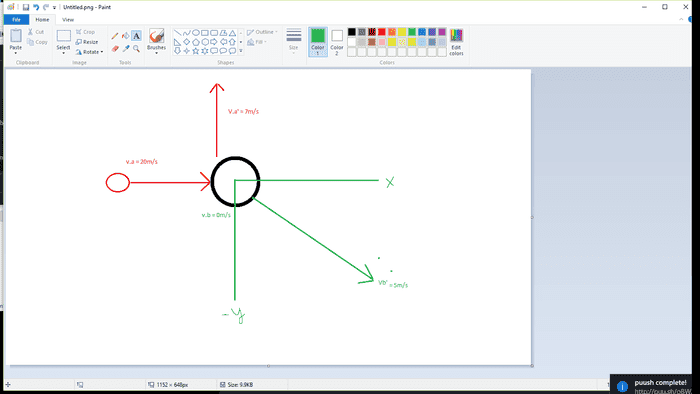# How to tell if energy was conserve in a momentum problem?

How can you determine if energy was conserve in a momentum problem? Let's say a small mass "m" hits a larger stationary mass "5m" where the smaller mass "m" flies bounces upward and the larger mass "5m" bounces in a negative downward direction.

So from them bouncing off each other I know that it's an elastic type of collision. What I also know is that the initial velocity of both masses are not the same after impact.

Can any of these clues allow me to tell if energy was conserve within the system?

Note: The picture is just something I made up and the values of the different velocities just demonstrate that the smaller ball loses speed after impact.

Does that mean that energy is not conserved since the smaller ball losses speed and therefore it losses kinetic energy? Does this make it an elastic collision instead of a perfectly elastic one?No, you cannot tell from the information you present.
If you know both velocities before and after collision, of course you can check if the KE is conserved.
If not, you need to know something about the materials. The quantity that tells "how ellastic" is the collision is called restitution coefficient. You can gind it for various pairs of materials.

No, you cannot tell from the information you present.
If you know both velocities before and after collision, of course you can check if the KE is conserved.
If not, you need to know something about the materials. The quantity that tells "how ellastic" is the collision is called restitution coefficient. You can gind it for various pairs of materials.

So if energy is conserved then the speed before impact should equal the speed after impact?

No, not at all. Where did you get this from? And what speed are you talking about? There are two speeds after a two-body collision.
And two speeds before collision. What would you mean by "the speed after impact should equal the speed after impact"?

No, not at all. Where did you get this from? And what speed are you talking about? There are two speeds after a two-body collision.
And two speeds before collision. What would you mean by "the speed after impact should equal the speed after impact"?

I mean the speed of the smaller ball (red). I thought that if the speed of the smaller ball before the impacted is different from the speed of the smaller ball after the impact are not the same value then the kinetic energy is not constant, therefore it's energy is not conserved. I think that my reasoning and definitions are wrong then.